Published: 10 October 2014

# Bearings damage detection in presence of heavy non-Gaussian noise via cyclo-stationary analysis

Jakub Obuchowski2
Agnieszka Wyłomańska3
1, 2Diagnostics and Vibro-Acoustic Science Laboratory, Wroclaw University of Technology,, Wroclaw, Poland
3Hugo Steinhaus Center, Institute of Mathematics and Computer Science, Wroclaw University of Technology, Wroclaw, Poland
Corresponding Author:
Views 30

#### Abstract

A problem of rolling element bearings diagnostics is discussed in the paper. In the classical approach, time domain detectors (e.g. kurtosis) or frequency domain representation (envelope spectrum analysis) are used to identify a damage in bearings. However, in our case, the machine (copper ore crusher) produces randomly spaced in time heavy impulsive disturbances related to normal operation of the machine. It eliminates completely possibility of detection of damage in time domain or using analysis of the envelope spectrum of the raw vibration signal. It is well known that effectiveness of envelope analysis might be significantly improved if the signal is pre-processed before enveloping. Unfortunately, again, almost all known criteria based on maximization of impulsiveness of signal in time domain fail in this case. In the paper we propose to incorporate cyclostationarity instead of impulsiveness, namely we propose to perform the spectral coherence density analysis.

## 1. Introduction

A problem of rolling element bearings diagnostics is discussed in the paper. In the classical approach, time domain detectors (e.g. kurtosis) [1, 3-9] or frequency domain representation (envelope spectrum analysis ) are used to identify damage in bearings . However, for damage detection at early stages , when signal to noise ratio is poor/informative signal is weak , damage detection becomes difficult and requires advanced signal processing including decomposition, modeling, adaptive filtering, etc. [10-14]. In our case, the machine (copper ore crusher) produces randomly spaced in time heavy impulsive disturbances related to normal operation of the machine, similarly as reported in [15-16]. It makes impossible detection of damage in time domain, moreover due to very poor signal to noise ratio, even envelope spectrum analysis is not able to detect damage directly based on raw vibration. It is well known that effectiveness of envelope analysis might be significantly improved if signal is pre-processed before enveloping. Unfortunately, impulsiveness cannot be basis for signal extraction anymore. So, the commonly used kurtosis-based optimization of pre-filtering (kurtogram, spectral kurtosis) cannot be applied here. Moreover, it is known that damage signature depends on load applied to the machine. Thus we claim that these non-periodic, strong impulses are non-informative from diagnostic point of view and should be separated from informative part. After that, informative, cyclic impulses related to the damaged part of REB should be analysed with taking into account operating condition. However, first step is to detect if there is any damage. Promising approach is to search for cyclic/periodic nature of impulses [17-20]. An envelope analysis without pre-filtering do not provide acceptable results, so we focused on cyclo-stationary approach, namely spectral coherence density map [16-20].

## 2. Machine and experiment description

The machine discussed here is a heavy duty crusher used in a mineral processing plant. The crusher is a kind of a machine which use a metal surface to crumble materials into small fractional pieces. During this process, as well as during entering material stream into the crusher, a lot of impacts/shocks appear. They are present in vibration signal acquired from the bearing’s housing. Several crushers were investigated. Vibration from bearings housing in horizontal and vertical direction and tacho signal have been measured using Endevco accelerometers Bruel Kjaer Laser probe, NI DAQ card and Labview Signal Express. Signals were acquired for idle mode as well as for different values of load (volume and granulation of material stream) with approx 120 s duration each. Sampling frequency was set to 25 kHz.

Fig. 1A crusher – general view (note bearings with yellow housing)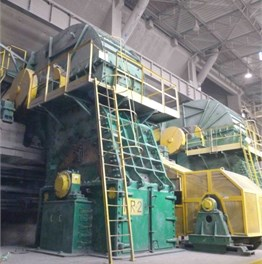## 3. Preliminary analysis

As mentioned, crushers are operating under non-stationary operating conditions that results in different values of amplitudes as well as frequency structure of acquired signals (see Figs. 2, 3).

It is impossible to recognize fault based on time series or time-frequency map due to number of impulses related to crushing process other “normal” operation of the machine. As it was mentioned, due to difficulties with automatic and even manual (based on STFT) informative frequency band selection, an envelope analysis was performed without pre-filtering. Fig. 4 shows envelope and its spectrum. Based on documentation one should expect signature at fault frequency equal to 30 Hz. From Fig. 4 it is difficult to recognize the damage.

## 4. Application of spectral coherence density for detection of cyclic impulsive disturbance in presence of non-Gaussian impulsive noise

The vibration signal from faulty bearings, so also our considered signals here are expected to exhibit the so called second-order cyclostationarity property (also called periodically correlated property, PC). Let us mention, the periodically correlated random processes of second-order are random systems in which there exists the periodic rythm in the structure that is generally more complicated than periodicity in the mean function of given signal . We say the signal $x\left(t\right)$ is cyclostationary with period $T$ if for each $s$ and $t$ the following properties are satisfied :

1
$\begin{array}{l}m\left(t\right)=E\left\{x\left(t\right)\right\}=m\left(t+T\right),\\ {R}_{x}\left(t,\tau \right)=E\left\{x\left(t\right)x\left(t-\tau {\right)}^{*}\right\}={R}_{x}\left(t+T,\tau \right).\end{array}$

And there are no smaller values of $T>$0 for which the above conditions hold.

The periodically correlated processes posses very interesting properties. Therefore they found many practical applications like in hydrology , meteorology , economics  and electrical engineering .

Fig. 2Raw vibration signal a) whole observation and b) part of the signal – without and with load applied (top/bottom respectively)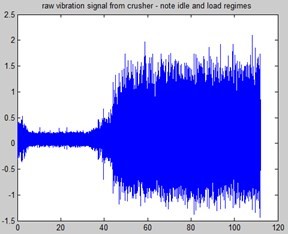a)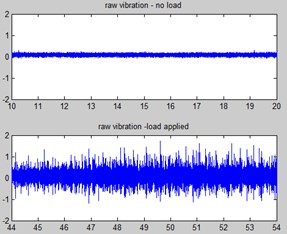b)

Fig. 3Time-Frequency map (STFT) of vibration signal idle mode a) and b) loaded machine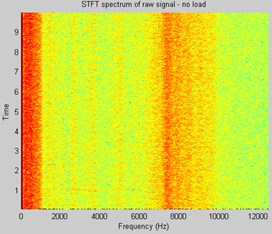a)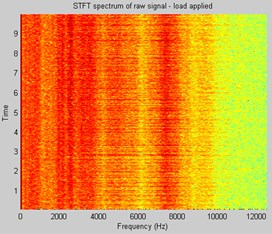b)

Fig. 4Envelope and envelope spectrum of part of the signal (load was applied)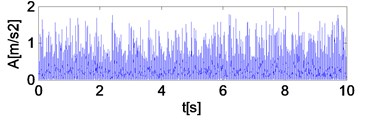a) Envelope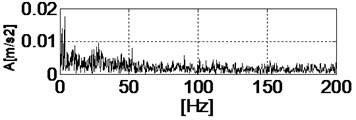b) Envelope spectrum of raw signal

Let us mention, one of the model that is can be used to description of the periodically correlated processes is periodic autoregressive moving average model (PARMA) which is an alternative to classical ARMA models uesd to description of stationary signals, .

If the signal $x\left(t\right)$ is considered to be second-order cyclostationary then, according to the definition of PC process, the autocorrelation function:

2
$\begin{array}{c}{R}_{x}\left(t,\tau \right)=E\left\{x\left(t\right)x\left(t-\tau {\right)}^{\mathrm{*}}\right\}\end{array},$

is periodic with respect to $t$ and then it has a Fourier series expansion:

3
${R}_{x}\left(t,\tau \right)={\sum }_{{\alpha }_{i}\in Α}{R}_{x}^{{\alpha }_{i}}\left(\tau \right)\cdot {e}^{j2\pi {\alpha }_{i}t},$

where the Fourier coefficients ${R}_{x}^{{\alpha }_{i}}\left(\tau \right)$ are known as the cyclic autocorrelation functions.

The Fourier transform of the cyclic autocorrelation function at a given cyclic frequency ${\alpha }_{i}$ then defines the cyclic power spectrum (CPS):

4
${S}_{x}^{{\mathrm{\alpha }}_{i}}\left(f\right)=F\left\{{R}_{x}^{{\mathrm{\alpha }}_{i}}\left(\tau \right)\right\}.$

If one consider set of CPS’s for all ${\alpha }_{i}\in A$, then we can get Spectral Correlation Density Map (SCDM):

5
$S{C}_{x}^{\alpha }\left(f\right)={\sum }_{{\alpha }_{i}\in Α}{S}_{x}^{{\alpha }_{i}}\left(f\right)\delta \left(\alpha -{\alpha }_{i}\right).$

In order to get a normalized version of SCDM it was proposed to use so called Spectral Coherence Map (SCM):

6
${\gamma }_{x}^{\alpha }=\frac{S{C}_{x}^{\alpha }\left(f\right)}{\sqrt{S{C}_{x}^{0}\left(f+\alpha /2\right)S{C}_{x}^{0}\left(f-\alpha /2\right)}}.$

As shown in Fig. 5 at frequency 30 Hz and its 2nd and 3rd harmonics vertical lines appeared. They correcponds to damage signature. So, one might conclude that using SCDM representation detection of the damage is possible. Moreover there are also other excitations that are not identified at this stage. It will be a task for furhter research.

It is clear that non-identified components mask damage signature. As reported in , there is a chance to „enhance“ SCD Map, as well as to extract signal of interest using advanced blind filtering algorithms .

Fig. 5Spectral coherence density map (note excitation at 30 Hz and its harmonics)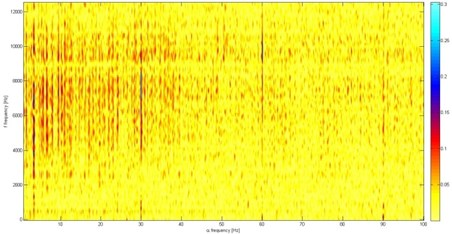## 5. Conclusions

In the paper preliminary results regarding application of Spectral Coherence Density for detection of cyclic impulsive disturbance in presence of non-Gaussian impulsive noise are presented. The vibration signals come from the crusher – a machine used in a mineral processing plant for pre-processing of minerals. During operation of the machine a lot of impacts are generated due to falling down of material stream and and the crushing process. Thus, detection of damaged bearings is very difficult. It has been proved that known time domain indicators, time-frequency representation or even comonly used envelope spectrum fail. The Spectral Coherence Density allows to detect modulating frequency alpha and its harmonics that correspond to the bearing‘s fault frequency. Further research will be focused on extraction of the signal of interest.